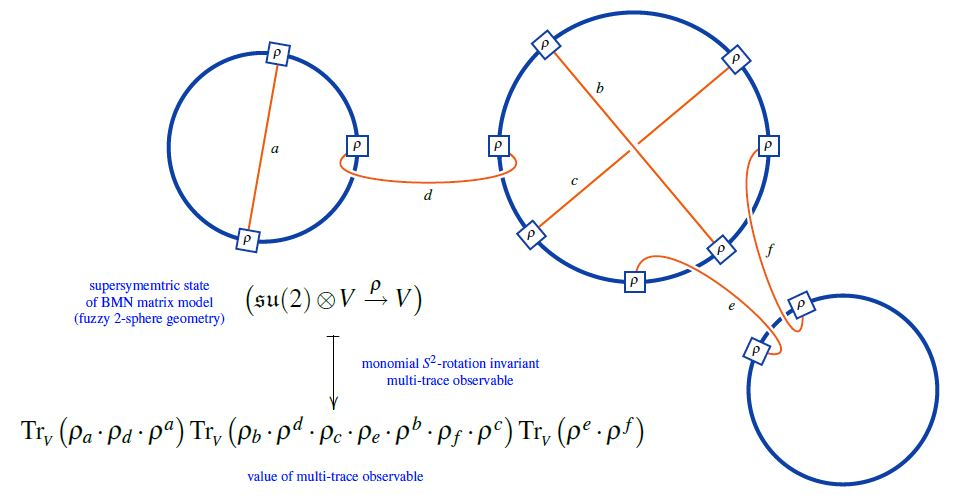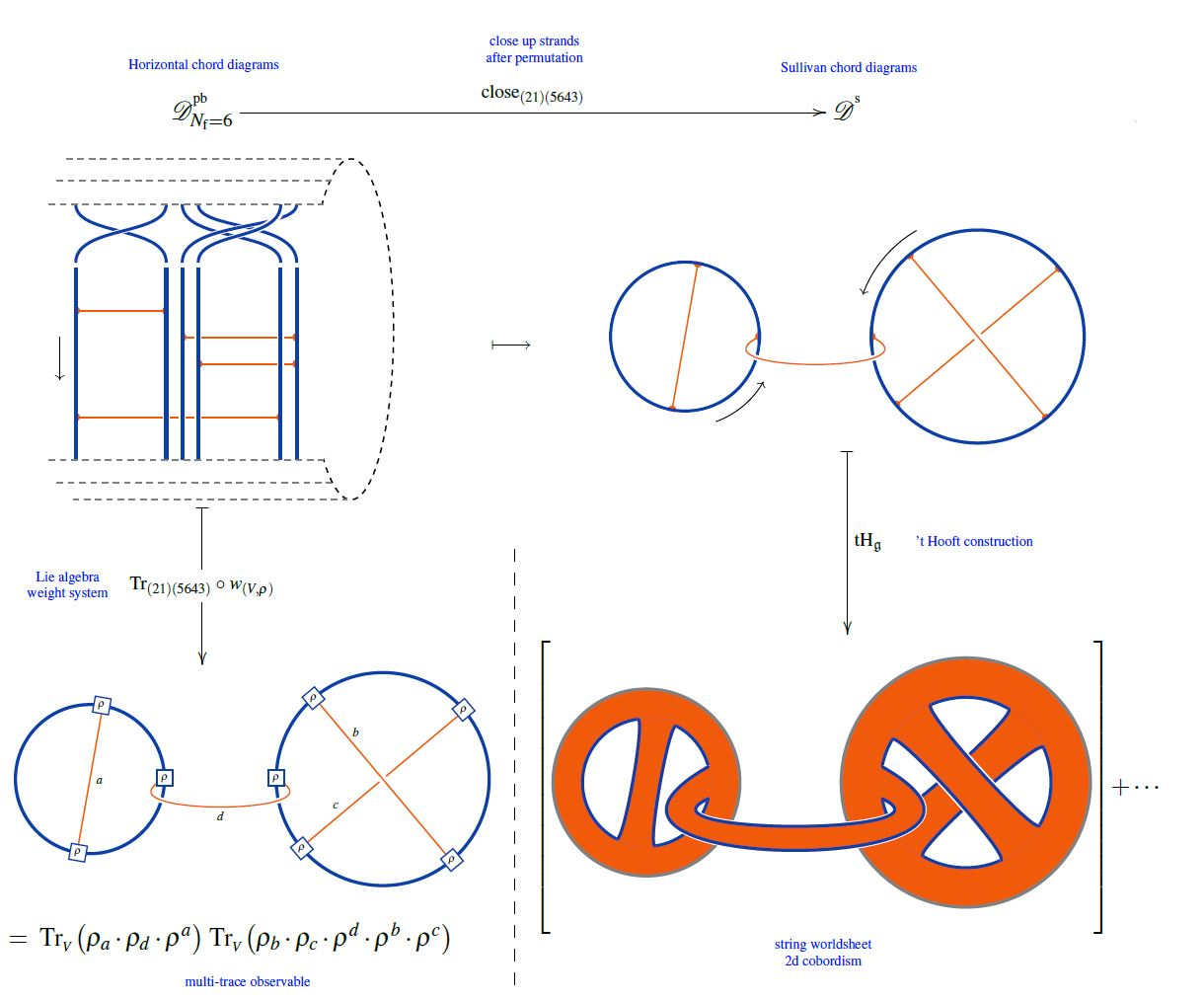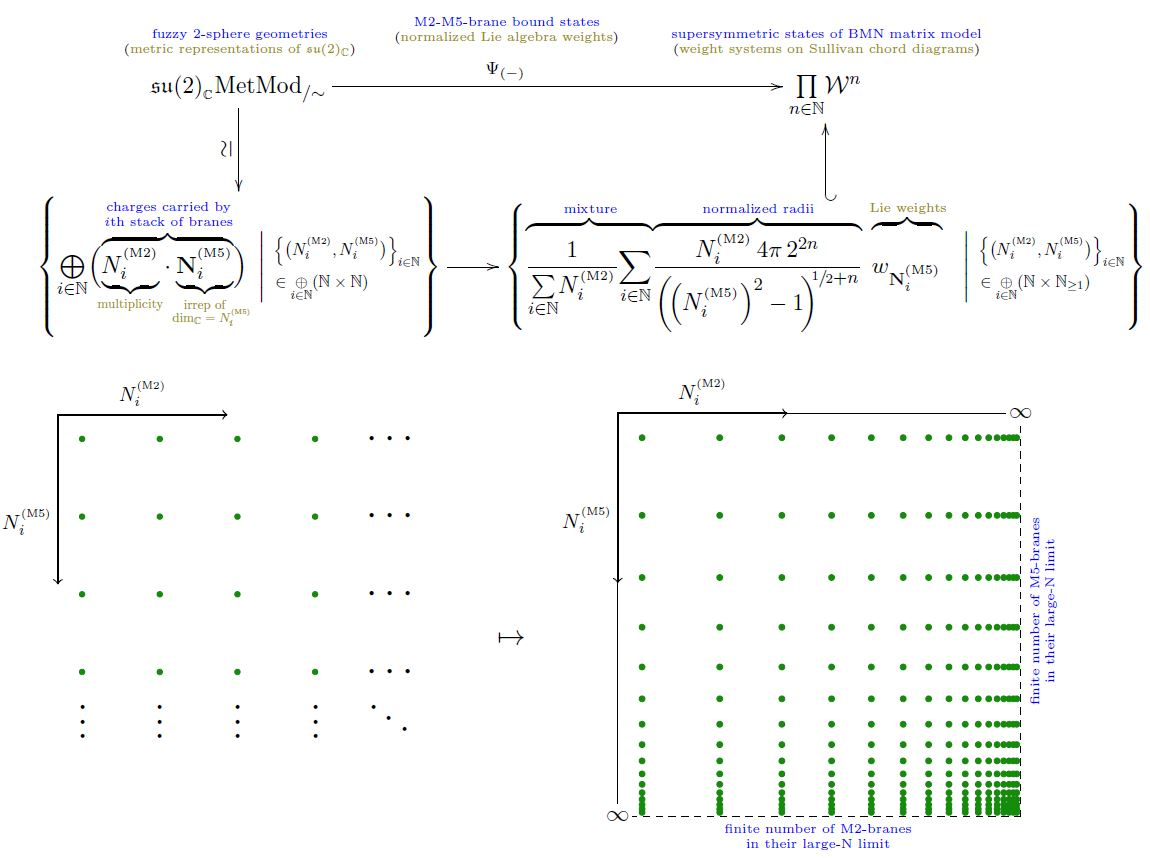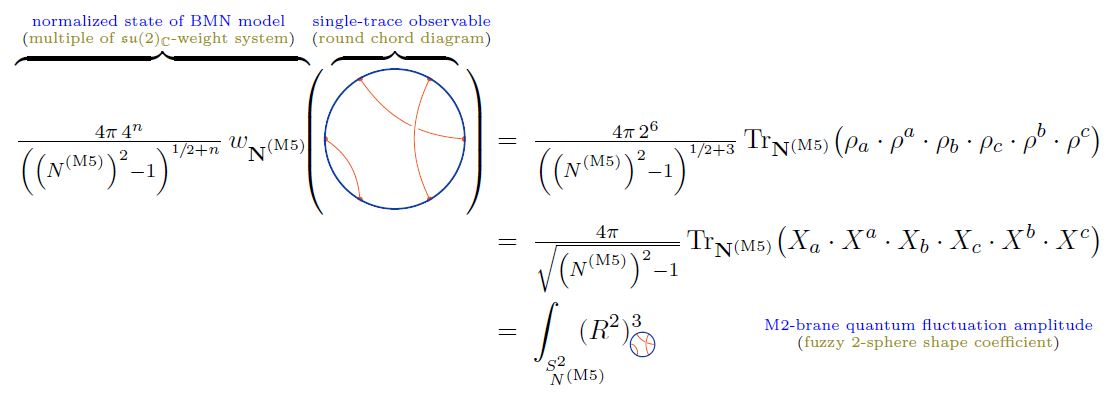Contents

# Contents

## Idea

The BMN matrix model (BMN 02, DSJVR 02) is a deformation of the BFSS matrix model by mass-terms and by a Chern-Simons-term. It arises as the light-cone gauge quantization of the M2-brane not on Minkowski spacetime as for the BFSS matrix model, but on its deformation to a pp-wave spacetime the mass-terms in the model are proportional to the B-field flux density in the pp-wave vacuum.

## Properties

### Ground states: $D0\perp D2$ and $M2/M5$ bound states

While the BFSS matrix model notoriously suffers from issues with the definition of its ground state (see there) this problem is fixed in the BMN matrix model by the mass term:

The supersymmetric classical solutions are configurations of fuzzy 2-spheres, corresponding to M2-brane-giant gravitons (BMN 02 (5.4), DSJVR 02, Section 4.2), which under the duality between M-theory and type IIA string theory become D0-D2 brane bound states (Lin04).

In fact, dually these gound states encode also transversal M5-branes and M2-M5 brane bound states, see below.

### Relation to black M2/M5-brane backgrounds

The pp-wave spacetime-background for the BMN matrix model arises as the Penrose limit (Inönü-Wigner contraction) of the AdS4x7-sphere near horizon geometry of both the black M2-brane spacetime $AdS_4 \times S^7$, as well as the AdS7$\times$4-sphere near horizon geometry of black M5-branes (BMN 02, Section 2).

### Chord diagrams as multi-trace observables in the BMN matrix model

The supersymmetric states of the BMN matrix model are temporally constant complex matrices which are complex metric Lie representations $\mathfrak{g} \otimes V \overset{\rho}{\to} V$ of $\mathfrak{g}=$su(2) (interpreted as fuzzy 2-sphere noncommutative geometries of giant gravitons or equivalently as fuzzy funnels of D0-D2 brane bound states).

A fuzzy 2-sphere-rotation invariant multi-trace observable on these supersymmetric states is hence an expression of the following form:Here we are showing the corresponding string diagram/Penrose notation for metric Lie representations, which makes manifest that

1. these multi-trace observables are encoded by Sullivan chord diagrams $D$

2. their value on the supersymmetric states $\mathfral{su}(2) \otimes V \overset{\rho}{\to}V$ is the evaluation of the corresponding Lie algebra weight system $w_{{}_V}$ on $D$.

Or equivalently, if $\widehat D$ is a horizontal chord diagram whose $\sigma$-permuted closure is $D$ (see here) then the values of the invariant multi-trace observables on the supersymmetric states of the BMN matrix model are the evaluation of $w_{V,\sigma}$ on $\widehat D$, as shown here:But since all horizontal weight systems are partitioned Lie algebra weight systems this way, this identifies supersymmetric states of the BMN matrix model as seen by invariant multi-trace observables as horizontal chord diagrams evaluated in Lie algebra weight systems.

### M2-M5 brane bound states in the BMN matrix model

There is the suggestion (MSJVR 02, checked in AIST 17a, AIST 17b) that, in the BMN matrix model, supersymmetric M2-M5-brane bound states are identified with isomorphism classes of certain “limit sequences” of longitudinal-light cone-constant $N \times N$-matrix-fields constituting finite-dimensional complex Lie algebra representations of su(2).

Concretely, if

$\underset{ i }{\oplus} \big( N^{(M2)}_i \cdot \mathbf{N}^{(M5)}_i \big) \;\;\in\;\; \mathfrak{su}(2)_{\mathbb{C}}Rep^{fin}$

denotes the representation containing

of the

• $N^{(M5)}_i$-dimensional irrep $\mathbf{N}^{(M5)}_i \in \mathfrak{su}(2)_{\mathbb{C}}Rep$

(for $\{N^{(M2)}_i, N^{(M5)}_i\}_{i} \in (\mathbb{N} \times \mathbb{N})^I$ some finitely indexed set of pairs of natural numbers)

with total dimension

$N \;\coloneqq\; dim \big( \underset{ i }{\oplus} \big( N^{(M2)}_i \cdot \mathbf{N}^{(M5)}_i \big) \big)$

then:

1. a configuration of a finite number of stacks of coincident M5-branes corresponds to a sequence of such representations for which

1. $N^{(M2)}_i \to \infty$ (this being the relevant large N limit)

2. for fixed $N^{(M5)}_i$ (being the number of M5-branes in the $i$th stack)

3. and fixed ratios $N^{(M2)}_i/N$ (being the charge/light-cone momentum carried by the $i$th stack);

2. an M2-brane configuration corresponds to a sequence of such representations for which

1. $N^{(M5)}_i \to \infty$ (this being the relevant large N limit)

2. for fixed $N^{(M2)}_i$ (being the number of M2-brane in the $i$th stack)

3. and fixed ratios $N^{(M5)}_i/N$ (being the charge/light-cone momentum carried by the $i$th stack)

for all $i \in I$.

Hence, by extension, any other sequence of finite-dimensional $\mathfrak{su}(2)$-representations is a kind of mixture of these two cases, interpreted as an M2-M5 brane bound state of sorts.

#### Formalization via weight systems on chord diagrams

To make this precise, let

$\mathfrak{su}(2)_{\mathbb{C}} MetMod_{/\sim} \;\in\; Set$

be the set of isomorphism classes of complex metric Lie representations (hence finite-dimensional representations) of su(2) (hence of the special linear Lie algebra $\mathfrak{sl}(2,C)$) and write

$Span \big( \mathfrak{su}(2)_{\mathbb{C}} MetMod_{/\sim} \big) \;\in\; Vect_{\mathbb{C}}$

Finally, write

$\array{ Span \big( \mathfrak{su}(2)_{\mathbb{C}}MetMod_{/\sim} \big) & \longrightarrow & \big( \mathcal{W}^{{}^{s}} \big)^{deg} \\ c_1 \cdot V_1 + c_2 \cdot V_2 &\mapsto& c_1 \cdot w_{V_1} + c_2 \cdot w_{V_2} }$

for the linear map which sends a formal linear combination or representations to the weight system on Sullivan chord diagrams with $deg \in \mathbb{N}$ chords which is given by tracing in the given representation.

Then a M2-M5-brane bound state as in the traditional discussion above, but now formalized as an su(2)-weight system

$\Psi_{ \left\{ N^{(M2)}_i, N^{(M5)}_i \right\}_{i} } \;\in\; \underset{ deg \in \mathbb{N} }{\prod} \big( \mathcal{W}^{{}^{\mathrm{s}}} \big)^{deg}$

hence a weight system horizontal chord diagrams closed to Sullivan chord diagrams, these now being the multi-trace observables on these these) is

(1)$\Psi_{ \left\{ N^{(M2)}_i, N^{(M5)}_i \right\}_{i} } \;\coloneqq\; \tfrac{ 4 \pi \, 2^{2\,deg} }{ \sum_i N^{(M2)}_i } \underset{i}{\sum} \left( N^{(M2)}_i \cdot \tfrac{1}{ \left( \sqrt{ \left( N^{(M5)}_i \right)^2 - 1 } \right)^{1 + 2 deg} } \mathbf{N}^{(M5)}_i \right)$graphics from Sati-Schreiber 19c

Normalization and large $N$ limit. The first power of the square root in (1) reflects the volume measure on the fuzzy 2-sphere (by the formula here), while the power of $2\,deg$ (which is the number of operators in the multi-trace observable evaluating the weight system) gives the normalization (here) of the functions on the fuzzy 2-sphere.

Hence this normalization is such that the single-trace observables among the multi-trace observables, hence those which come from round chord diagrams, coincide on those M2-M5 brane bound states $\Psi_{ \left\{ N^{(M2)}_i, N^{(M5)}_i \right\}_{i} }$ for which $N^{(M2)}_i = \delta_i^{i_0} N^{(M2)}$, hence those which have a single constitutent fuzzy 2-sphere, with the shape observables on single fuzzy 2-spheres discussed here:graphics from Sati-Schreiber 19c

Therefore, with this normalization, the limits $N^{(M2)} \to \infty$ and $N^{(M5)} \to \infty$ of (1) should exist in weight systems. The former trivially so, the latter by the usual convergence ofthe fuzzy 2-sphere to the round 2-sphere in the large N limit.

Notice that the multi trace observables on these states only see the relative radii of the constitutent fuzzy 2-spheres: If $N^{(M2)}_i = \delta_i^{i_0} N^{(M2)}$ then the $N^{(M2)}$-dependence of (1) cancels out, reflecting the fact that then there is only a single constituent 2-sphere of which the observable sees only the radius fluctuations, not the absolute radius (proportional to $N^{(M2)}$).

### General

The original articles:

Review:

In relation to the Myers effect and D0-D2 brane bound states:

Discussion of the large N limit:

• Corneliu Sochichiu, Continuum limit(s) of BMN matrix model: Where is the (nonabelian) gauge group?, Phys.Lett. B574 (2003) 105-110 (arXiv:hep-th/0206239)

• Jens Hoppe. Ki-Myeong Lee, New BPS Configurations of BMN Matrix Theory, JHEP 0806:041, 2008 (arXiv:0712.3616)

• Masanori Hanada, Lorenzo Mannelli, Yoshinori Matsuo, Four-dimensional $\mathcal{N}=1$ super Yang-Mills from matrix model, Phys. Rev. D 80, 125001 (arXiv:0905.2995)

• Yolanda Lozano, Carlos Nunez, Salomon Zacarias, BMN Vacua, Superstars and Non-Abelian T-duality, JHEP 09 (2017) 008 (arXiv:1703.00417)

• Yuhma Asano, Samuel Kováčic, Denjoe O’Connor, The Confining Transition in the Bosonic BMN Matrix Model (arXiv:2001.03749)

### Thermodynamics

Discussion of thermodynamics, phase transitions, confinement and thermal field theory of the BMN matrix model:

• Hyeonjoon Shin, Kentaroh Yoshida, Thermodynamic Behavior of Fuzzy Membranes in PP-Wave Matrix Model, Phys.Lett. B627 (2005) 188-196 (arXiv:hep-th/0507029)

• Curtis T. Asplund, David Berenstein, Diego Trancanelli, Evidence for fast thermalization in the plane-wave matrix model, Phys. Rev. Lett. 107, 171602 (2011) (arXiv:1104.5469)

• Lucas Brady, Vatche Sahakian, Scrambling with Matrix Black Holes, Phys Rev D 88, 046003 (2013) (arXiv:1306.5200)

• Miguel S. Costa, Lauren Greenspan, Joao Penedones, Jorge Santos, Thermodynamics of the BMN matrix model at strong coupling, JHEP 03 (2015) 069 (arXiv:1411.5541)

• Samuel Pramodh, Vatche Sahakian From Black Hole to Qubits: Evidence of Fast Scrambling in BMN theory, JHEP 7, July 2015, 67 (arXiv:1412.2396)

• Yuhma Asano, Veselin G. Filev, Samuel Kováčik, Denjoe O’Connor, The non-perturbative phase diagram of the BMN matrix model, JHEP 1807 (2018) 152 (arXiv:1805.05314)

• Yuhma Asano, Samuel Kováčic, Denjoe O’Connor, The Confining Transition in the Bosonic BMN Matrix Model (arXiv:2001.03749)

Discussion of chaos in the M2-brane physics described by the BMN matrix model:

• Minos Axenides, Emmanuel Floratos, Georgios Linardopoulos, M2-brane Dynamics in the Classical Limit of the BMN Matrix Model, Phys. Lett. B773 (2017) 265 (arXiv:1707.02878)

### KK-compactification from $D=4$$\mathcal{N}=4$ SYM

On the BMN matrix model as a KK-compactification of D=4 N=4 super Yang-Mills theory on the 3-sphere:

Using the KK-compactification of D=4 N=4 super Yang-Mills theory to the BMN matrix model for lattice gauge theory-computations in D=4 N=4 SYM and for numerical checks of the AdS-CFT correspondence:

• Masazumi Honda, Goro Ishiki, Sang-Woo Kim, Jun Nishimura, Asato Tsuchiya, Direct test of the AdS/CFT correspondence by Monte Carlo studies of N=4 super Yang-Mills theory, JHEP 1311 (2013) 200 (arXiv:1308.3525)

### M5-branes in the BMN matrix model

The proposal of passing to horizontal/vertical limits of sizes of partitions is due to:

A detailed check is in:

• Yuhma Asano, Goro Ishiki, Shinji Shimasaki, Seiji Terashima, Spherical transverse M5-branes from the plane wave matrix model, JHEP 02 (2018) 076 (arXiv:1711.07681)

Review in:

• Yuhma Asano, Goro Ishiki, Shinji Shimasaki, Seiji Terashima, On the transverse M5-branes in matrix theory, Phys. Rev. D 96, 126003 (2017) (arXiv:1701.07140)